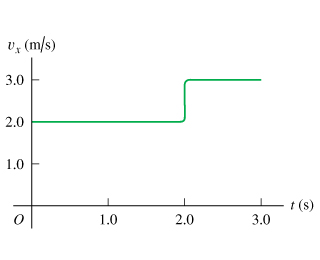# Problem: A ball moves in a straight line (the x-axis). The graph in the figure below shows this ball's velocity as a function of time. (a) What is the ball's average velocity during the first 2.8  s?(b) What is the ball's average speed during the first 2.8 s?(c) Suppose that the ball moved in such a way that the graph segment after 2.0  s was -3.0 m/s instead of +3.0 m/s. Find the ball's average velocity during the first 2.8  s in this case.(d) Find the ball's average speed during the first 2.8  s in the case described in part C.

###### FREE Expert Solution
88% (427 ratings)
###### Problem Details
A ball moves in a straight line (the x-axis). The graph in the figure below shows this ball's velocity as a function of time.
(a) What is the ball's average velocity during the first 2.8  s?
(b) What is the ball's average speed during the first 2.8 s?
(c) Suppose that the ball moved in such a way that the graph segment after 2.0  s was -3.0 m/s instead of +3.0 m/s. Find the ball's average velocity during the first 2.8  s in this case.
(d) Find the ball's average speed during the first 2.8  s in the case described in part C.